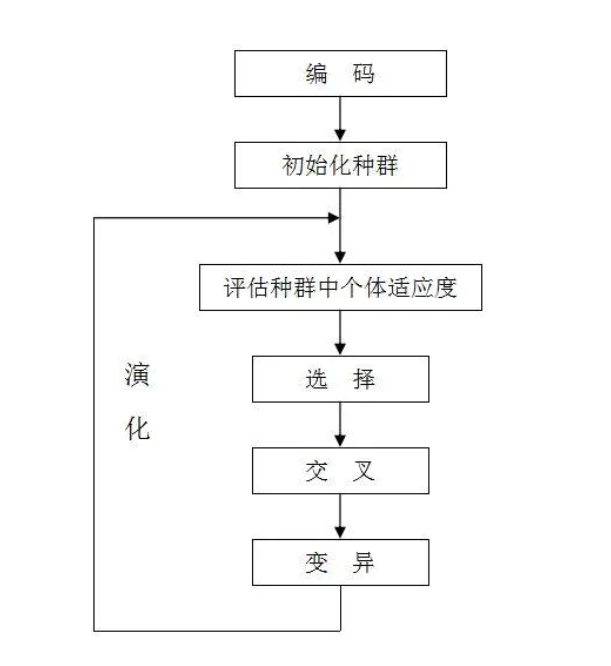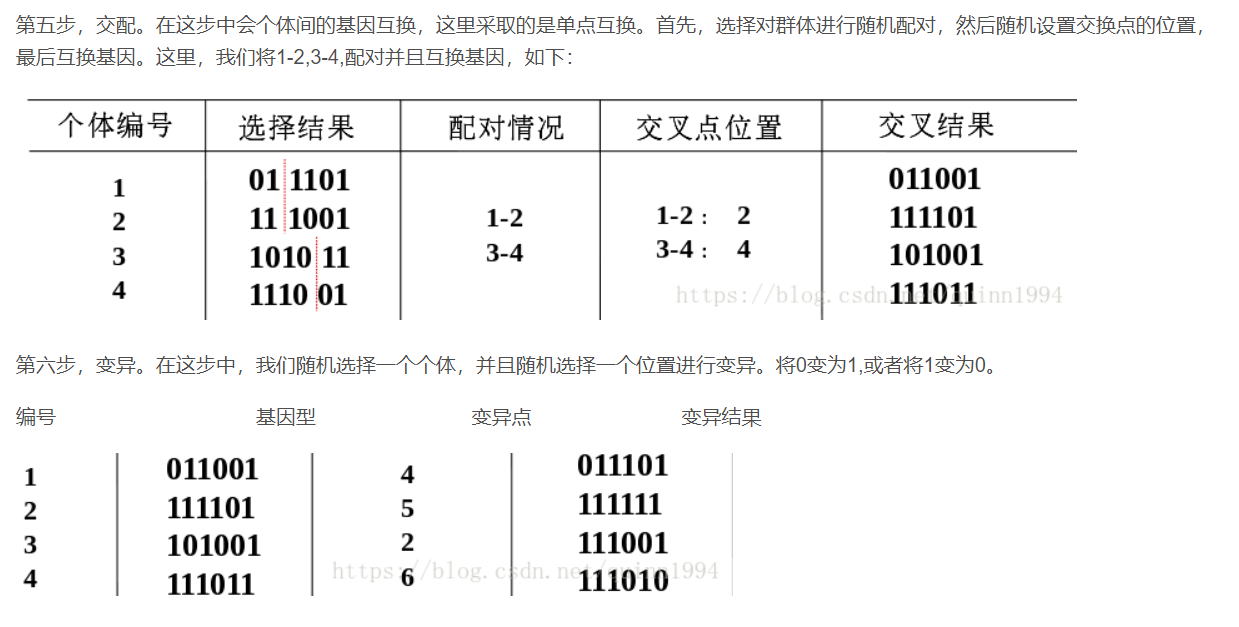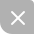# 二、深度学习模型+遗传算法

## 2.代码部分import numpy as np
import pandas as pd
import os

DNA_SIZE = 24   #编码的位数，越大越精确，但计算量越大
POP_SIZE = 200  #初始化种群数量
CROSSOVER_RATE = 0.8  #交叉率
MUTATION_RATE = 0.005  #变异率
N_GENERATIONS = 2   #迭代次数
X_BOUND = [10, 20]   #变量x的取值范围   阳极流速
Y_BOUND = [100, 500]  #变量y的取值范围   阴极流速


folder = "D:\Desktop\First"
os.chdir(folder)
global model


'''解码'''
def translateDNA(pop):  # pop表示种群矩阵，一行表示一个二进制编码表示的DNA，矩阵的行数为种群数目
x_pop = pop[:, 1::2]  # 奇数列表示X  [::2]表示隔一个取一个  [::-1]可视为翻转操作
y_pop = pop[:, ::2]  # 偶数列表示y

# pop:(POP_SIZE,DNA_SIZE)*(DNA_SIZE,1) --> (POP_SIZE,1)
x = x_pop.dot(2 ** np.arange(DNA_SIZE)[::-1]) / float(2 ** DNA_SIZE - 1) * (X_BOUND - X_BOUND) + X_BOUND
y = y_pop.dot(2 ** np.arange(DNA_SIZE)[::-1]) / float(2 ** DNA_SIZE - 1) * (Y_BOUND - Y_BOUND) + Y_BOUND
return x, y'''交叉变异'''
def crossover_and_mutation(pop, CROSSOVER_RATE=0.8):
new_pop = []
for father in pop:  # 遍历种群中的每一个个体，将该个体作为父亲
child = father  # 孩子先得到父亲的全部基因（这里我把一串二进制串的那些0，1称为基因）
if np.random.rand() < CROSSOVER_RATE:  # 产生子代时不是必然发生交叉，而是以一定的概率发生交叉
mother = pop[np.random.randint(POP_SIZE)]  # 再种群中选择另一个个体，并将该个体作为母亲
cross_points = np.random.randint(low=0, high=DNA_SIZE * 2)  # 随机产生交叉的点
child[cross_points:] = mother[cross_points:]  # 孩子得到位于交叉点后的母亲的基因
mutation(child)  # 每个后代有一定的机率发生变异
new_pop.append(child)

return new_pop

def mutation(child, MUTATION_RATE=0.003):
if np.random.rand() < MUTATION_RATE:  # 以MUTATION_RATE的概率进行变异
mutate_point = np.random.randint(0, DNA_SIZE*2)  # 随机产生一个实数，代表要变异基因的位置
child[mutate_point] = child[mutate_point] ^ 1  # 将变异点的二进制为反转


'''适应度函数'''
def F(x, y):
num = x.shape
print(num)
X2 = []
for i in range(num):
X = [x[i], y[i], 2200, 1000, 1000, 1000, 1000]
X2.append(X)
Xtest = np.array(X2)
X_test = Xtest.reshape(-1, 7)
Y_pre = model.predict(X_test)

return Y_pre[:, 0]

'''计算适应度'''
def get_fitness(pop):
x, y = translateDNA(pop)
pred = F(x, y)
return (pred - np.min(pred)) + 1e-3  #计算最大值 减去最小的适应度是为了防止适应度出现负数，通过这一步fitness的范围为[0, np.max(pred)-np.min(pred)],最后在加上一个很小的数防止出现为0的适应度
#return -(pred - np.max(pred)) + 1e-3 #计算最小值
'''


'''根据适应度进行选择'''
def select(pop, fitness):  # nature selection wrt pop's fitness
idx = np.random.choice(np.arange(POP_SIZE), size=POP_SIZE, replace=True, p=(fitness) / (fitness.sum()))
return pop[idx]


'''输出结果'''
def print_info(pop):
fitness = get_fitness(pop)
max_fitness_index = np.argmax(fitness)
print("max_fitness:", fitness[max_fitness_index])
x, y = translateDNA(pop)
print("最优的基因型：", pop[max_fitness_index])
print("(x, y):", (x[max_fitness_index], y[max_fitness_index]))

X = [x[max_fitness_index], y[max_fitness_index], 2200.0, 1000.0, 1000.0, 1000.0, 1000.0]
X = np.array(X)
XMAX = X.reshape(-1, 7)
Y_PRE = model.predict(XMAX)
Y_MAX = Y_PRE[:, 0]
print("最优化结果为：", Y_MAX)


# 三、完整代码

'''
autor: wsw
Time: 2021.4.24
'''
from tensorflow_core import keras
import numpy as np
import pandas as pd
import os

DNA_SIZE = 24   #编码的位数，越大越精确，但计算量越大
POP_SIZE = 200  #初始化种群数量
CROSSOVER_RATE = 0.8  #交叉率
MUTATION_RATE = 0.005  #变异率
N_GENERATIONS = 2   #迭代次数
X_BOUND = [10, 20]   #变量x的取值范围   阳极流速
Y_BOUND = [100, 500]  #变量y的取值范围   阴极流速

folder = "D:\Desktop\First"
os.chdir(folder)
global model

'''适应度函数'''
def F(x, y):
num = x.shape
print(num)
X2 = []
for i in range(num):
X = [x[i], y[i], 2200, 1000, 1000, 1000, 1000]
X2.append(X)
Xtest = np.array(X2)
X_test = Xtest.reshape(-1, 7)
Y_pre = model.predict(X_test)

return Y_pre[:, 0]

'''计算适应度'''
def get_fitness(pop):
x, y = translateDNA(pop)
pred = F(x, y)
return (pred - np.min(pred)) + 1e-3  #计算最大值 减去最小的适应度是为了防止适应度出现负数，通过这一步fitness的范围为[0, np.max(pred)-np.min(pred)],最后在加上一个很小的数防止出现为0的适应度
#return -(pred - np.max(pred)) + 1e-3 #计算最小值
'''

'''

'''解码'''
def translateDNA(pop):  # pop表示种群矩阵，一行表示一个二进制编码表示的DNA，矩阵的行数为种群数目
x_pop = pop[:, 1::2]  # 奇数列表示X  [::2]表示隔一个取一个  [::-1]可视为翻转操作
y_pop = pop[:, ::2]  # 偶数列表示y

# pop:(POP_SIZE,DNA_SIZE)*(DNA_SIZE,1) --> (POP_SIZE,1)
x = x_pop.dot(2 ** np.arange(DNA_SIZE)[::-1]) / float(2 ** DNA_SIZE - 1) * (X_BOUND - X_BOUND) + X_BOUND
y = y_pop.dot(2 ** np.arange(DNA_SIZE)[::-1]) / float(2 ** DNA_SIZE - 1) * (Y_BOUND - Y_BOUND) + Y_BOUND
return x, y

'''交叉变异'''
def crossover_and_mutation(pop, CROSSOVER_RATE=0.8):
new_pop = []
for father in pop:  # 遍历种群中的每一个个体，将该个体作为父亲
child = father  # 孩子先得到父亲的全部基因（这里我把一串二进制串的那些0，1称为基因）
if np.random.rand() < CROSSOVER_RATE:  # 产生子代时不是必然发生交叉，而是以一定的概率发生交叉
mother = pop[np.random.randint(POP_SIZE)]  # 再种群中选择另一个个体，并将该个体作为母亲
cross_points = np.random.randint(low=0, high=DNA_SIZE * 2)  # 随机产生交叉的点
child[cross_points:] = mother[cross_points:]  # 孩子得到位于交叉点后的母亲的基因
mutation(child)  # 每个后代有一定的机率发生变异
new_pop.append(child)

return new_pop

def mutation(child, MUTATION_RATE=0.003):
if np.random.rand() < MUTATION_RATE:  # 以MUTATION_RATE的概率进行变异
mutate_point = np.random.randint(0, DNA_SIZE*2)  # 随机产生一个实数，代表要变异基因的位置
child[mutate_point] = child[mutate_point] ^ 1  # 将变异点的二进制为反转

'''根据适应度进行选择'''
def select(pop, fitness):  # nature selection wrt pop's fitness
idx = np.random.choice(np.arange(POP_SIZE), size=POP_SIZE, replace=True, p=(fitness) / (fitness.sum()))
return pop[idx]

'''输出结果'''
def print_info(pop):
fitness = get_fitness(pop)
max_fitness_index = np.argmax(fitness)
print("max_fitness:", fitness[max_fitness_index])
x, y = translateDNA(pop)
print("最优的基因型：", pop[max_fitness_index])
print("(x, y):", (x[max_fitness_index], y[max_fitness_index]))

X = [x[max_fitness_index], y[max_fitness_index], 2200.0, 1000.0, 1000.0, 1000.0, 1000.0]
X = np.array(X)
XMAX = X.reshape(-1, 7)
Y_PRE = model.predict(XMAX)
Y_MAX = Y_PRE[:, 0]
print("最优化结果为：", Y_MAX)

if __name__ == "__main__":
pop = np.random.randint(2, size=(POP_SIZE, DNA_SIZE * 2))  # matrix (POP_SIZE, DNA_SIZE)
for _ in range(N_GENERATIONS):  # 迭代N代
x, y = translateDNA(pop)   #解码
pop = np.array(crossover_and_mutation(pop, CROSSOVER_RATE))   #交叉变异
# F_values = F(translateDNA(pop), translateDNA(pop))#x, y --> Z matrix
fitness = get_fitness(pop)   #计算适应度
pop = select(pop, fitness)  # 选择生成新的种群

print_info(pop)


# 四、总结UP更新不错过~被折叠的  条评论 为什么被折叠?到【灌水乐园】发言w^s^w

¥2 ¥4 ¥6 ¥10 ¥20余额支付 (余额：-- )扫码支付获取中扫码支付点击重新获取扫码支付1.余额是钱包充值的虚拟货币，按照1:1的比例进行支付金额的抵扣。
2.余额无法直接购买下载，可以购买VIP、C币套餐、付费专栏及课程。余额充值